Ex 5.1

Chapter 5 Class 12 Continuity and Differentiability
Serial order wise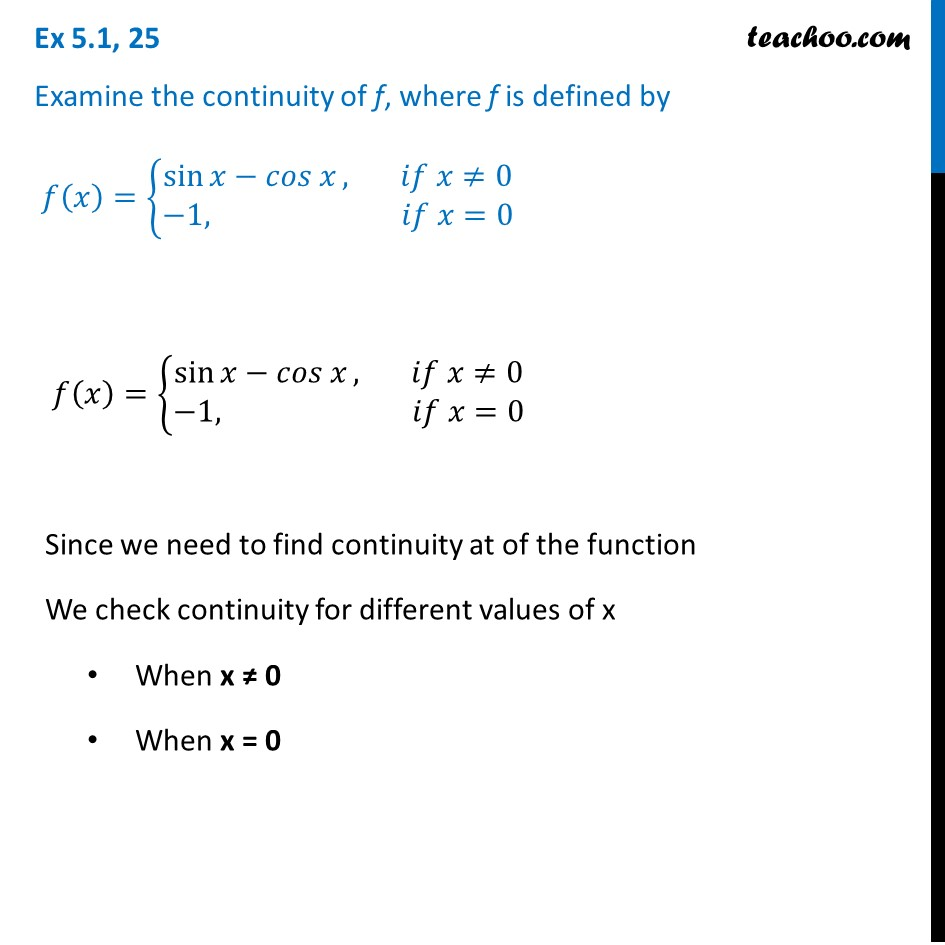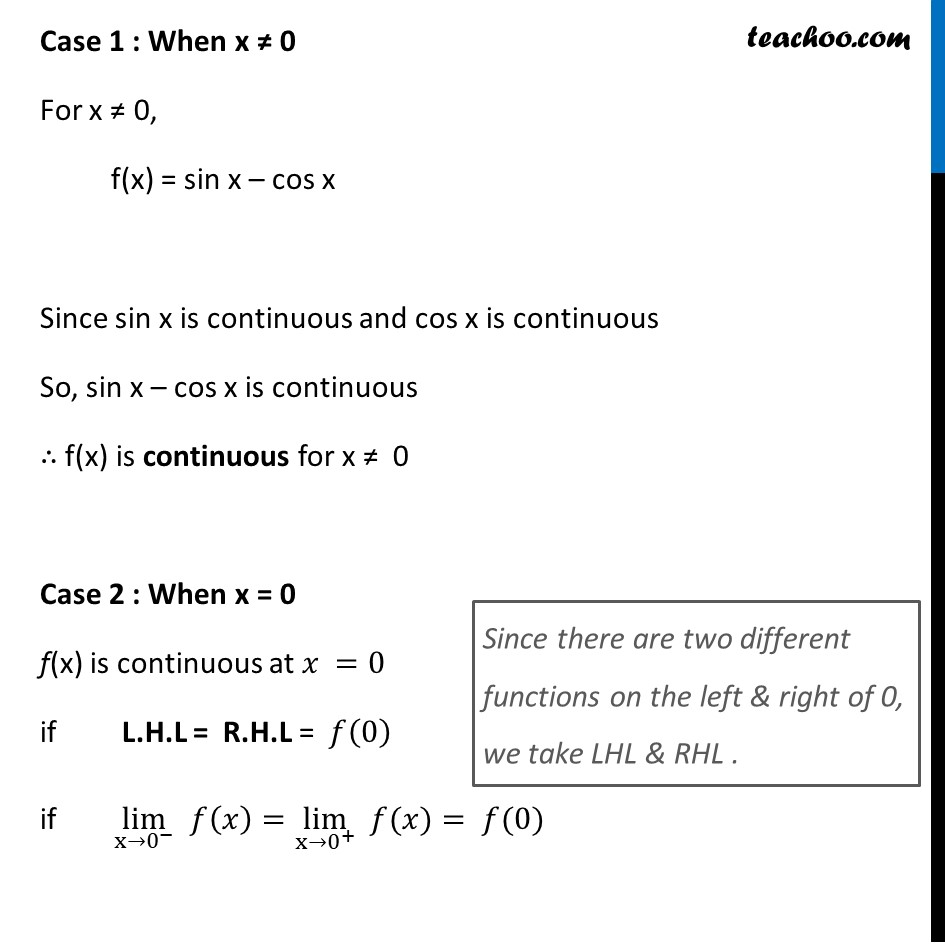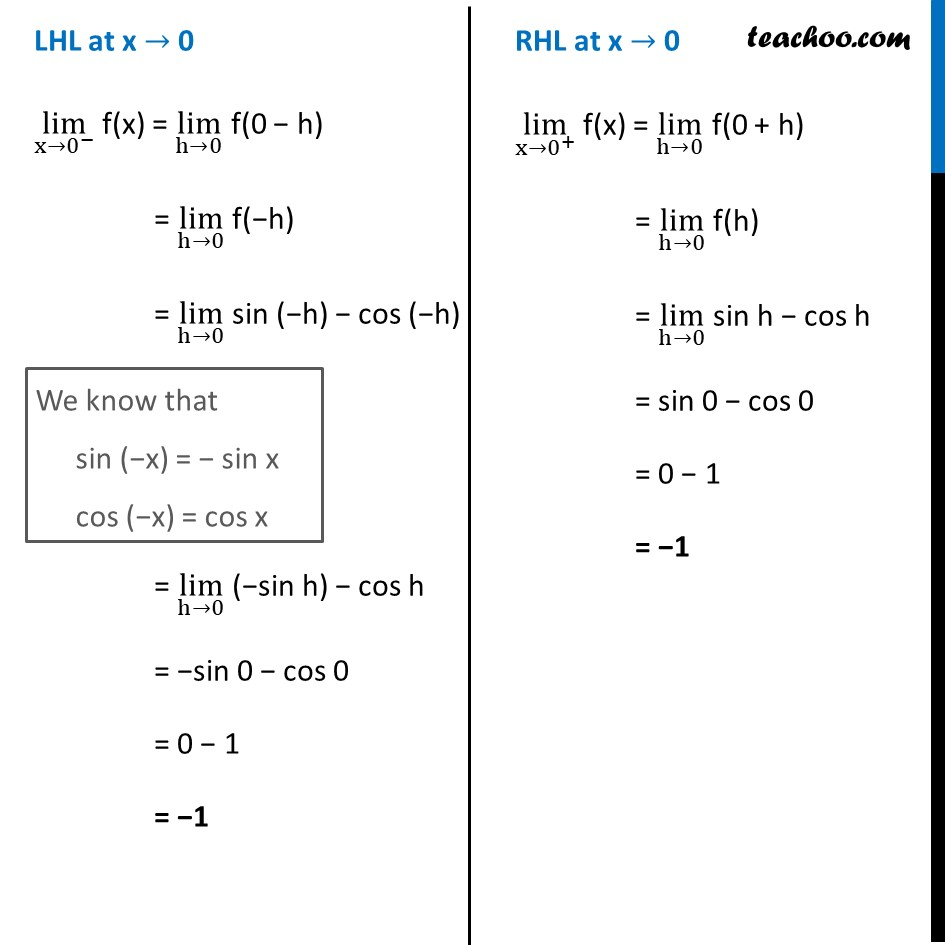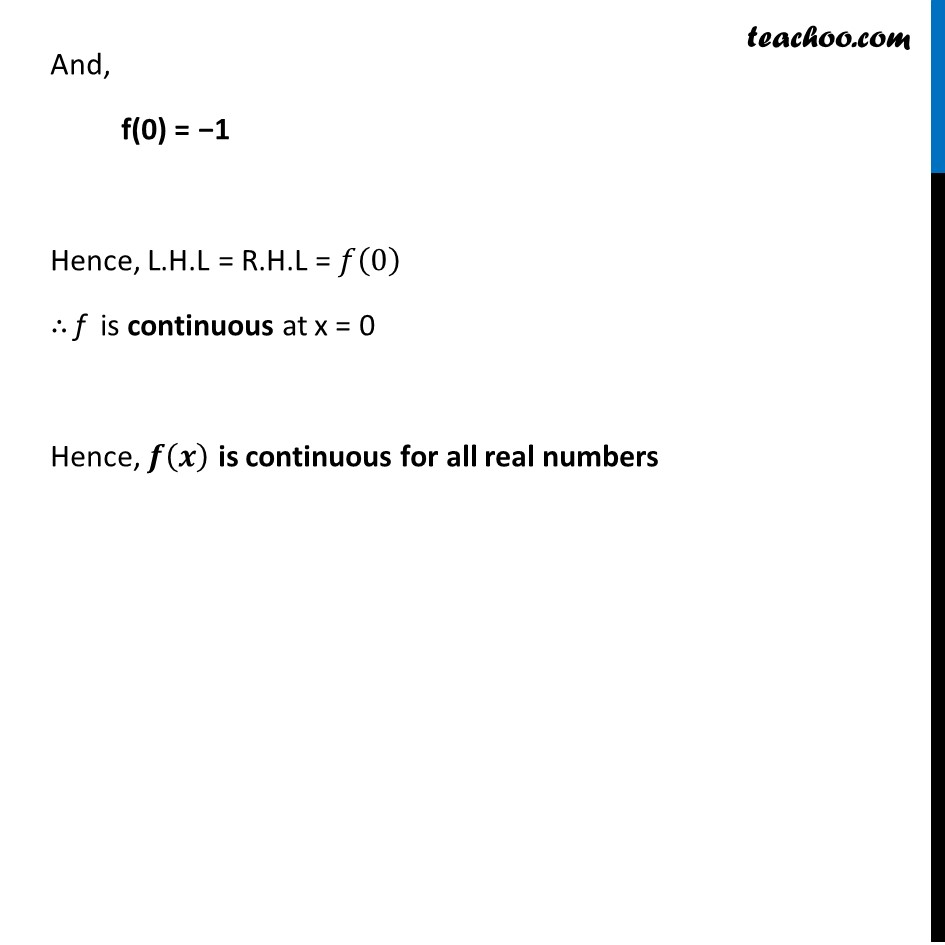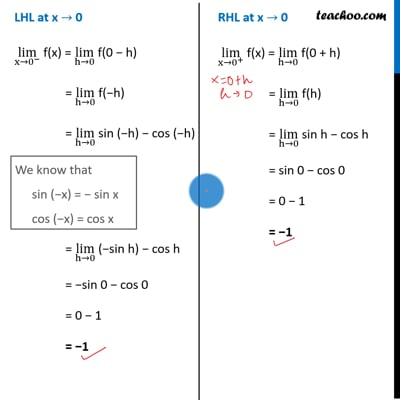This video is only available for Teachoo black users

Get live Maths 1-on-1 Classs - Class 6 to 12

### Transcript

Ex 5.1, 25 Examine the continuity of f, where f is defined by 𝑓(𝑥)={█(sin⁡〖𝑥−𝑐𝑜𝑠 𝑥〗, 𝑖𝑓 𝑥≠[email protected]&−1, 𝑖𝑓 𝑥=0)┤ 𝑓(𝑥)={█(sin⁡〖𝑥−𝑐𝑜𝑠 𝑥〗, 𝑖𝑓 𝑥≠[email protected]&−1, 𝑖𝑓 𝑥=0)┤ Since we need to find continuity at of the function We check continuity for different values of x When x ≠ 0 When x = 0 Case 1 : When x ≠ 0 For x ≠ 0, f(x) = sin x – cos x Since sin x is continuous and cos x is continuous So, sin x – cos x is continuous ∴ f(x) is continuous for x ≠ 0 Case 2 : When x = 0 f(x) is continuous at 𝑥 =0 if L.H.L = R.H.L = 𝑓(0) if lim┬(x→0^− ) 𝑓(𝑥)=lim┬(x→0^+ ) " " 𝑓(𝑥)= 𝑓(0) Since there are two different functions on the left & right of 0, we take LHL & RHL . LHL at x → 0 lim┬(x→0^− ) f(x) = lim┬(h→0) f(0 − h) = lim┬(h→0) f(−h) = lim┬(h→0) sin (−h) − cos (−h) = lim┬(h→0) (−sin h) − cos h = −sin 0 − cos 0 = 0 − 1 = −1 We know that sin (−x) = − sin x cos (−x) = cos x RHL at x → 0 lim┬(x→0^+ ) f(x) = lim┬(h→0) f(0 + h) = lim┬(h→0) f(h) = lim┬(h→0) sin h − cos h = sin 0 − cos 0 = 0 − 1 = −1 And, f(0) = −1 Hence, L.H.L = R.H.L = 𝑓(0) ∴ f is continuous at x = 0 Hence, 𝒇(𝒙) is continuous for all real numbers